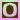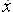Go to content
The following applet can be used to illustrate how the chain rule applies to the derivative of f(g(x)) at x=a. Simply enter the functions g(x) and f(x) and the value a. The applet automatically draws the graphs of g(x), f(x) and f(g(x)). The appropriate line tangent to the graph is drawn in red. For g(x), the tangent line goes through the point (a,g(a)), for f(x), the tangent line goes through the point (g(a),f(g(a))) and for f(g(x)), the tangent line goes through the point (a,f(g(a))). The value of a can be changed by simply typing a new value, such as "1.2345", "pi/2", "sqrt(5)+cos(3)", etc. You may also change this value by using the up/down arrow keys or dragging the corresponding point left or right. To move the center of the graph, simply drag any point to a new location. To label the x-axis in radians (i.e. multiples of pi), click on the graph and press "control-r". To switch back, simply press "control-r" again.This page uses an applet from David Little (Lecturer in the Mathematics Department, Penn State University, USA) and is used with his permission.

## Glossary

### chain rule

A rule for differentiating a function of a function:
dy/dx = dy/du x du/dx.

### derivative

rate of change, dy/dx, f'(x),, Dx.

### graph

A diagram showing a relationship between two variables.
The diagram shows a vertical y axis and a horizontal x axis.

### rule

A method for connecting one value with another.

### tangent

1. The trigonometrical function defined as opposite/adjacent in a right-angled triangle.
2. A straight line that touches a curve at one point.

Full Glossary List

## This question appears in the following syllabi:

SyllabusModuleSectionTopicExam Year
AP Calculus AB (USA)3DifferentiationRules - the chain rule-
AP Calculus BC (USA)3DifferentiationRules - the chain rule-
AQA A-Level (UK - Pre-2017)C3DifferentiationRules - the chain rule-
AQA A2 Maths 2017Pure MathsDifferentiationChain Rule-
AQA AS/A2 Maths 2017Pure MathsDifferentiationChain Rule-
CBSE XII (India)CalculusContinuity and DifferentiabilityDerivative of composite functions, chain rule-
CBSE XI (India)CalculusLimits and DerivativesSum, difference, product and quotient rules-
CCEA A-Level (NI)C3DifferentiationRules - the chain rule-
CIE A-Level (UK)P2DifferentiationRules - the chain rule-
Edexcel A-Level (UK - Pre-2017)C3DifferentiationRules - the chain rule-
Edexcel A2 Maths 2017Pure MathsDifferentiationChain Rule-
Edexcel AS/A2 Maths 2017Pure MathsDifferentiationChain Rule-
I.B. Higher Level6DifferentiationRules - the chain rule-
I.B. Standard Level6DifferentiationRules - the chain rule-
Methods (UK)M8DifferentiationRules - the chain rule-
OCR A-Level (UK - Pre-2017)C3DifferentiationRules - the chain rule-
OCR A2 Maths 2017Pure MathsDifferentiation TechniquesChain Rule-
OCR MEI A2 Maths 2017Pure MathsDifferentiation TechniquesChain Rule-
OCR-MEI A-Level (UK - Pre-2017)C3DifferentiationRules - the chain rule-
Pre-U A-Level (UK)4DifferentiationRules - the chain rule-
Scottish Advanced HighersM1DifferentiationRules - the chain rule-
Scottish (Highers + Advanced)HM3DifferentiationRules - the chain rule-
Scottish HighersM3DifferentiationRules - the chain rule-
Universal (all site questions)DDifferentiationRules - the chain rule-
WJEC A-Level (Wales)C3DifferentiationRules - the chain rule-